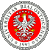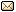XXXIX Workshop on Geometric Methods in Physics 27.06-3.07.2021 IX School on Geometry and Physics 21-25.06.2021
 Home First Announcement Travel and local information Lecturers (WGMP) Lecturers (SGP) Registration Photos Proceedings Previous Workshops Email

# Hitoshi Konno

## Elliptic stable envelopes and finite-dimensional representation of the elliptic quantum group $U_{q,p}(\widehat{sl}_N)$

We present a construction of elliptic stable envelopes for partial flag variety as elliptic weight functions of type $gl_N$ using representation theory of the elliptic quantum group $U_{q,p}(\widehat{sl}_N)$. We also present a finite-dimensional representation of $U_{q,p}(\widehat{sl}_N)$ on the Gelfand-Tsetlin basis. The result is described in a combinatorial way in terms of the partitions of $[1,n]$, and gives an elliptic and dynamical analogue of the geometric representation of the quantum affine algebra $U_q(\widehat{sl}_N)$ on equivariant K-theory constructed by Ginzburg-Vasserot and Nakajima. The elliptic stable envelopes allows us to obtain a geometric interpretation of our result as a representation of $U_{q,p}(\widehat{sl}_N)$ on equivariant elliptic cohomology.

 Event sponsored by: University of BialystokWebpage by: Tomasz Golinski